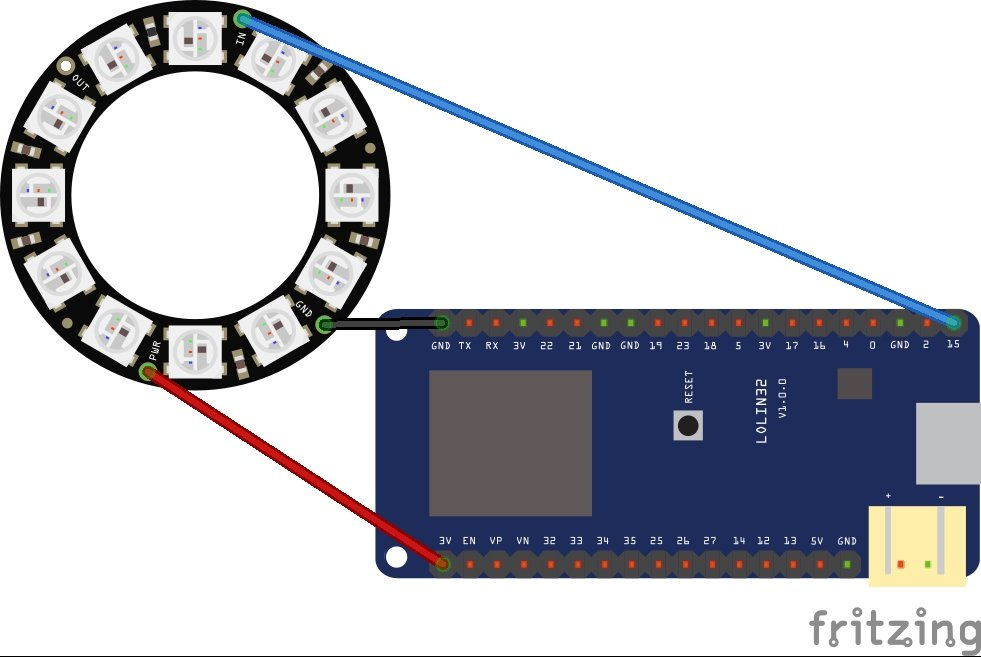# Random colours on the WS2812b led ring

This was a WS2812b led ring that I purchased for low cost. There are 12 LEDs in total on this and once you solder 3 cables to the module you are ready to go

We will create an example for the Lolin32 using the Arduino IDEConnect Vcc to Wemos Lolin 3.3 volts, Gnd to Gnd and the In to 15, you can change this to any pin but you will need to change the code below

Here is a little layout so you get the ideaesp32 and led ring

2 examples which display random colours on the leds

```#include <Adafruit_NeoPixel.h>

#define PIN 15
// How many NeoPixels are attached to the Arduino?
#define NUMPIXELS 12

Adafruit_NeoPixel pixels = Adafruit_NeoPixel(NUMPIXELS, PIN, NEO_GRB + NEO_KHZ800);

int delayval = 250; // delay for half a second

void setup()
{
pixels.begin(); // This initializes the NeoPixel library.
randomSeed(analogRead(0));
}

void loop()
{

// pixels.Color takes RGB values, from 0,0,0 up to 255,255,255
int led = random(12);
int redNumber = random(255);
int greenNumber = random(255);
int blueNumber = random(255);
pixels.setPixelColor(i, pixels.Color(redNumber,greenNumber,blueNumber));
pixels.show(); // This sends the updated pixel color to the hardware.
delay(delayval); // Delay for a period of time (in milliseconds).
}
```
```#include <Adafruit_NeoPixel.h>

#define PIN 15
// How many NeoPixels are attached to the Arduino?
#define NUMPIXELS 12

Adafruit_NeoPixel pixels = Adafruit_NeoPixel(NUMPIXELS, PIN, NEO_GRB + NEO_KHZ800);

int delayval = 250; // delay for half a second

void setup()
{
pixels.begin(); // This initializes the NeoPixel library.
randomSeed(analogRead(0));
}

void loop()
{
for(int i=0;i<NUMPIXELS;i++)
{
// pixels.Color takes RGB values, from 0,0,0 up to 255,255,255
int redNumber = random(255);
int greenNumber = random(255);
int blueNumber = random(255);
pixels.setPixelColor(i, pixels.Color(redNumber,greenNumber,blueNumber));
pixels.show(); // This sends the updated pixel color to the hardware.
delay(delayval); // Delay for a period of time (in milliseconds).
}

}
```